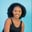Related Tags

numpy
python3

# How to use the numpy.gradient function for a 2D array in PythonMaria Elijah

## Overview

We can use the numpy.gradient() function to find the gradient of an N-dimensional array. For gradient approximation, the function uses either first or second-order accurate one-sided differences at the boundaries and second-order accurate central differences in the interior (or non-boundary) points.

Note: To create a two-dimensional (2D) array in Python, we can use a list of lists.

### Syntax

numpy.gradient(f, *varargs, axis=None, edge_order=1)

### Parameters

• f: This is the N-dimensional array containing scalar function samples for which gradient will calculate the gradient.
• varargs: This is an optional parameter that represents a scalar list. It contains the sample distances for each dimension—dx, dy, dz, and so on—total N scalars. 1 is the default value for this argument.
• axis: This is an optional parameter representing the axis along which gradient will calculate the gradient. If this is not None, then the number of varargs must equal the axes. The default value for this argument is None.
• edge_order: {1,2} This is an optional parameter.
• gradient: This will calculate the gradient using Nth order (as specified by edge_order) accurate boundary differences. The default value for this argument is 1.

### Return value

The numpy.gradient() method returns an ndarray or a list of ndarrays representing the gradient.

Note: The result for 2D arrays will be two arrays ordered by axis.

### Example

The following code shows how to use the numpy.gradient() method for 2D arrays.

# import numpy
import numpy as np
# create list
x1 = [7, 4, 8, 3]
x2 = [2, 6, 5, 9]
# convert the lists to 2D array using np.array
f = np.array([x1, x2])

# compute the gradient of an N-dimensional array
# and store the result in result

print(result)
Using the np.floor_divide() function

### Explanation

• Line 2: We import the numpy library.
• Lines 4–5: We create two lists, x1 and x2.
• Line 7: We use the np.array() method to transform the lists into a 2D array and store it in f.
• Line 11: We compute the gradient of f using the np.gradient() function and store it in result.
• Line 13: We print result.

RELATED TAGS

numpy
python3

CONTRIBUTORMaria Elijah
RELATED COURSES

View all Courses

Keep Exploring

Learn in-demand tech skills in half the time